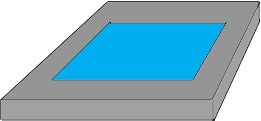SEARCH HOMEMath Central Quandaries & QueriesQuestion from Jerri: I have a rectangular pool 15x30 and it will require stone placed around it 4 feet out and 4 inches deep how much will I need? Thanks, jerriHi Jerri,

I drew a sketch of the pool and stone (not to scale).The pool is 15 feet by 30 feet and the stone region is 4 feet wide all the way around so the external dimension of the pool and stone are $15 + 4 + 4 = 23$ feet by $30 + 4 + 4 = 38$ feet. You can find the volume you want by first finding the volume of a rectangular region which is 23 feet by 38 feet by 4 inches and then removing the volume of a region that is 15 feet by 30 feet by 4 inches. Both of these volumes you can calculate by using our volume calculator.

Write back if you need more assistance,
PennyMath Central is supported by the University of Regina and the Imperial Oil Foundation.